# Guest post by Amir Ali Ahmadi: Sum of Squares (SOS) Techniques: An Introduction, Part II/II

The reader is encouraged to read Part I of this series before reading this post. For ease of reference, we recall the polynomial optimization problem from our previous post:

(1)This is the task of minimizing a multivariate polynomial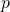over a basic semialgebraic setdefined by the polynomials.

Sum of squares and semidefinite programming

If a polynomial is nonnegative, can we write it in a way that its nonnegativity becomes obvious? This is the meta-question behind Hilbert’s 17th problem. As the title of this post suggests, one way to achieve this goal is to try to write the polynomial as a sum of squares of polynomials. We say that a polynomialis a sum of squares (sos), if it can be written asfor some polynomials. Existence of an sos decomposition is an algebraic certificate for nonnegativity. Remarkably, it can be decided by solving a single semidefinite program.

Theorem 1 A multivariate polynomialin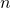variables and of degree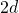is a sum of squares if and only if there exists a positive semidefinite matrix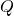(often called the Gram matrix) such that

(2)whereis the vector of monomials of degree up to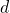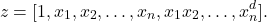The proof is straightforward and is left to the reader. Note that the feasible set defined by these constraints is the intersection of an affine subspace (arising from the equality constraints matching the coefficients ofwith the entries ofin (2)) with the cone of positive semidefinite matrices. This is precisely the semidefinite programming (SDP) problem. The size of the Gram matrixis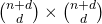, which for fixedis polynomial in. Depending on the structure of, there are well-documented techniques for further reducing the size of the Gram matrixand the monomial vector. We do not pursue this direction here but state as an example that ifis homogeneous of degree, then it suffices to place in the vectoronly monomials of degree exactly.

Example: Consider the task proving nonnegativity of the polynomialSince this is a form (i.e., a homogeneous polynomial), we take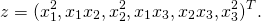One feasible solution to the SDP in (2) is given by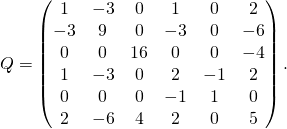Upon a decomposition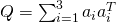, with,,, one obtains the sos decomposition

(3)You are probably asking yourself right now whether every nonnegative polynomial can be written as a sum of squares. Did we just get lucky on the above example? Well, from complexity considerations alone (see previous post), we know that we should expect a gap between nonnegative and sos polynomials, at least for large.

In a seminal 1888 paper, Hilbert was the first to show that there exist nonnegative polynomials that are not sos. In fact, for each combination of degree and dimension, he showed whether such polynomials do or do not exist. For example, one famous theorem of Hilbert from that paper is that all nonnegative ternary quartic forms are sos. So we did not really use any luck in our example above! The same would have happened for any other nonnegative quartic form in three variables. Hilbert’s proof of existence of nonnegative polynomials that are not sos was not constructive. The first explicit example interestingly appeared nearly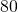years later and is due to Motzkin:

(4)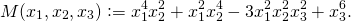Nonnegativity of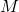follows from the arithmetic-geometric inequality (why?). Non-existence of an sos decomposition can be shown by some algebraic manipulations or by the systematic tools we have today for showing infeasibility of a semidefinite program (separating hyperplane theorems). From an application viewpoint, the good news for sum of squares optimization is that constructing polynomials of the type in (4) is not a trivial task. This is especially true if additional structure is required on the polynomial. For example, the following problem is still open.

Open problem. Construct an explicit example of a convex, nonnegative polynomial that is not a sum of squares.

This question is due to Parrilo. The motivation behind it is quite clear: we would like to understand whether sos optimization is exact for the special case of convex polynomial optimization problems. Blekherman has shown with non-constructive arguments that such “bad” convex polynomials must exist when the degree is four or larger and the number of variables goes to infinity. However, we do not know the smallest (or any reasonable) dimension for which this is possible and lack any explicit examples. For the reader interested in tackling this problem, it is known that any such convex polynomial must necessarily be not “sos-convex”. Roughly speaking, sos-convex polynomials are convex polynomials whose convexity is certified by an appropriately defined sum of squares identity. Examples of convex but not sos-convex polynomials have recently appeared and a characterization of the degrees and dimensions where they exist is now available. Interestingly, this characterization coincides with that of Hilbert, for reasons that are also not well-understood; see Chapter 3 of our thesis.

Having shown that not every nonnegative polynomial is a sum of squares of polynomials, Hilbert asked in his 17th problem whether every such polynomial can be written as a sum of squares of rational functions. Artin answered the question in the affirmative in 1927. As we will see next, such results allow for a hierarchy of semidefinite programs that approximate the set of nonnegative polynomials better and better.

Positivstellensatz and the SOS hierarchy

Consider proving a statement that we all learned in high school: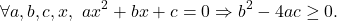Just for the sake of illustration, let us pull an algebraic identity out of our hat which certifies this claim:

(5)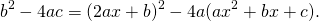Think for a second why this constitutes a proof. The Positivstellensatz is a very powerful algebraic proof system that vastly generalizes what we just did here. It gives a systematic way of certifying infeasibility of any system of polynomial equalities and inequalities over the reals. Sum of squares representations play a central role in it. (They already did in our toy example above if you think about the role of the first term on the right hand side of (5)). Modern optimization theory adds a wonderfully useful aspect to this proof system: we can now use semidefinite programming to automatically find suitable algebraic certificates of the type in (5).

The Positivstellensatz is an example of a theorem of the alternative. The reader may already be familiar with similar fundamental theorems, such as the Hilbert’s (weak) Nullstellensatz (1893),

a system of polynomial equations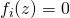is infeasible over the complex numbersthere exist polynomialssuch that,

or the Farkas Lemma (1902) of linear programming,

a system of linear (in)equalities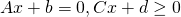is infeasible over the realsthere exist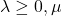such that.

These theorems typically have an “easy” (well, trivial) direction and a “hard” direction. The same is true for the Positivstellensatz.

Theorem 2  (Positivstellensatz — Stengle (1974)) The basic semialgebraic setis emptythere exist polynomialsand sum of squares polynomials,such that

(6)The number of terms in this expression is finite since we never raise any polynomial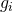to a power larger than one. The sum of squares polynomials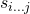are of course allowed to be the zero polynomial, and in practice many of them often are. There are bounds in the literature on the degree of the polynomials,, but of exponential size as one would expect for complexity reasons. There is substantial numerical evidence, however, from diverse application areas, indicating that in practice (whatever that means) the degrees of these polynomials are usually quite low. We remark that the Positivstellensatz is a very powerful result. For example, it is straightforward to show that the solution to Hilbert’s 17th problem follows as a corollary of this theorem (how?).

Under minor additional assumptions, refined versions of the Positivstellensatz we presented are available. The two most well-known are perhaps due to Schmüdgen and Putinar. For example, Putinar’s Positivstellensatz states that if the setsatisfies the so-called Archimedean property (a property slightly stronger than compactness), then emptiness ofguarantees a representation of the type (6), where the second and third line are scratched out; i.e., there is no need to take products of the constraints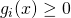. While this may look like a simplification at first, there is a tradeoff: the degree of the sos multipliersmay need to be higher in Putinar’s representation than in Stengle’s. This makes intuitive sense as the proof system needs to additionally prove statements of the type, while in Stengle’s representation this is taken as an axiom.

SOS hierarchies. Positivstellensatz results form the basis of sos hierarchies of Parrilo and Lasserre for solving the polynomial optimization problem (1). The two approaches only differ in the version of the Positivstellensatz they use (originally, Parrilo’s paper follows Stengle’s version and Lasserre’s follows Putinar’s), and the fact that Lasserre presents the methodology from the dual (but equivalent) viewpoint of moment sequences. In either case though, the basic idea is pretty simple. We try to obtain the largest lower bound for problem (1), by finding the largestfor which the set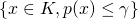is empty. We certify this emptiness by finding Positivstellensatz certificates. In levelof the hierarchy, the degree of the polynomialsand the sos polynomailsin (6) is bounded by. Asincreases, the quality of the lower bound monotonically increases, and for each fixed, the search for the optimal, and the polynomials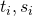is a semidefinite optimization problem (possibly with some bisection over).

Revisiting MAXCUT

Recall from Seb’s earlier lecture that we saw a beautiful algorithm for MAXCUT due to Goemans and Williamson which produced an approximation ratio of. The algorithm had two steps: first we solved a semidefinite program, then we performed some clever randomized rounding. In this section we focus only on the first step. We show that even low degree Positivstellensatz refutations can produce stronger bounds than the standard SDP relaxation.

Consider the 5-cycle with all edge weights equal to one. It is easy to see that the MAXCUT value of this graph is equal to. However, the standard SDP relaxation (i.e. the one used in the Goemans and Williamson algorithm) produces an upper bound of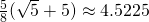.

The MAXCUT value of the 5-cycle is equal to minus the optimal value of the quadratic program

(7)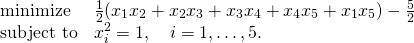We will find the largest constantsuch that the objective function minusis algebraically certified to be nonnegative on the feasible set. To do this, we solve the sos optimization problemThe decision variables of this problem are the constantand the coefficients of the polynomials, which in this case we parametrize to be quadratic functions. This sos program results in a polynomially sized semidefinite optimization problem via Theorem 1. The optimal value of the program is; i.e., we have solved the MAXCUT instance exactly.

You may be wondering, “can we show that a certain level of the sos hierarchy combined with an appropriately designed rounding procedure produces an approximation ratio of better than?” Let’s just say that if you did this, you would probably become an overnight celebrity.

Software

There are very nice implementations of sum of squares optimization solvers that automate the process of setting up the resulting semidefinite programs. The interested reader may want to play around with SOSTOOLS, YALMIP, or GloptiPoly.

Impact

While we focused in this post on the polynomial optimization problem, the impact of sum of squares optimization goes much beyond this area. In dynamics and control, sos optimization has enabled a paradigm shift from classical linear control to an efficient framework for design of nonlinear controllers that are provably safer, more agile, and more robust. Papers on applications of sos optimization have appeared in areas as diverse as quantum information theory, robotics, geometric theorem proving, formal verification, derivative pricing, stochastic optimization, and game theory, among others. In theoretical computer science, sos techniques are currently a subject of intense study. Not too long ago, I attended a plenary presentation at the CCC by Subhash Khot, where one of the last things he said was, “the sos hierarchies are currently the greatest threat to the unique games conjecture.” If you are an ORFE student and still not convinced that this SOS business is actually useful, you may find relief in knowing that SOS is in fact the core discipline of ORFE 😉

This entry was posted in Optimization. Bookmark the permalink.